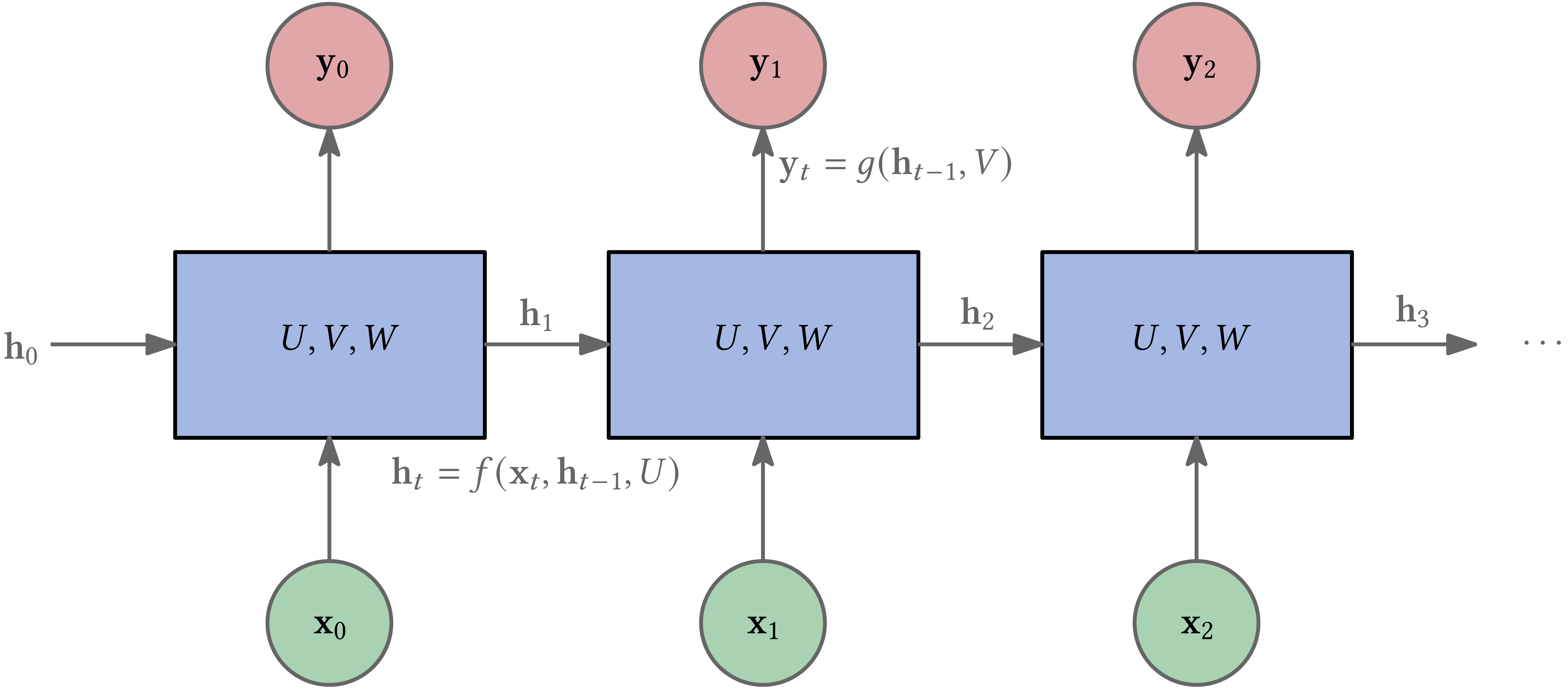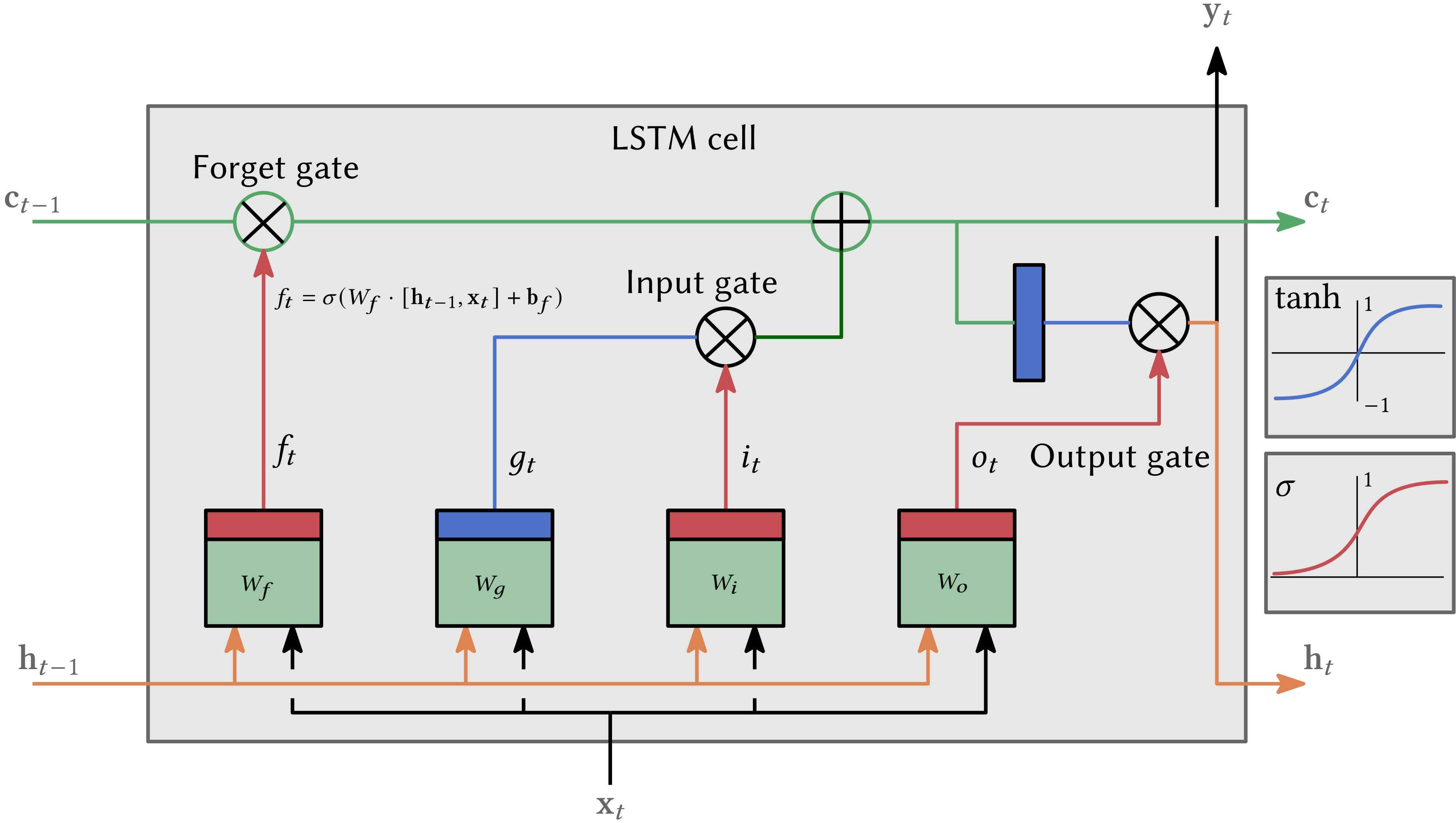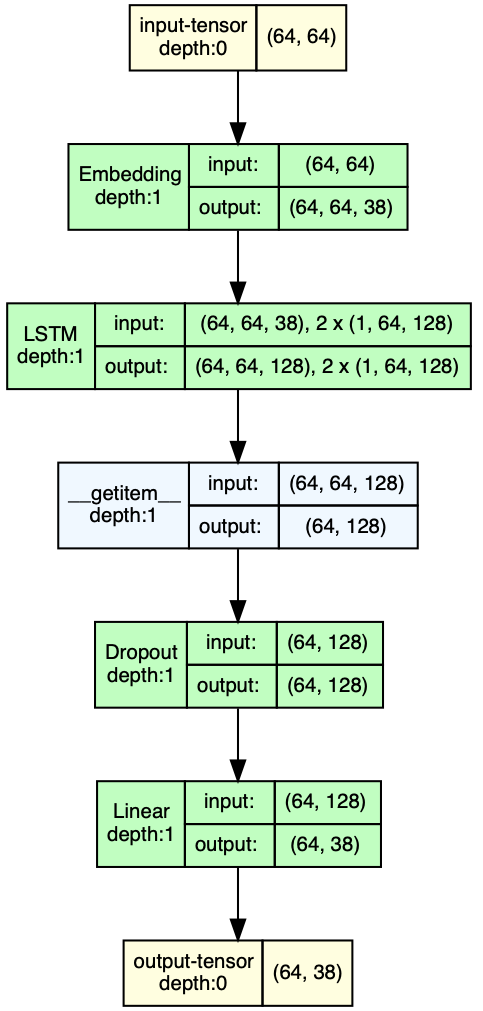This article is the continuation of my series Musical Interrogation. It is recommended that you read the these articles first. This time we use a recurrent neural network, more preceisly an LSTM which I explained a little bit in Introduction. An LSTM is a RNN that couter acts the problem of exploding and vanishing gradients.Figure 1: Sketch of an RNN unfolded in time.

The article gives some explanation to the code in the following notebook which can be executed on Google Colab. Because our model is now able to learn long-time relations, we can use the GridEncoder, i.e., a piano roll data representation which is exaclty what we do.

## Data Preparation

Again we use the data from EsAC. The specific dataset I utilized is Folksongs from the continent of Europe and for the purpose of this work, I will exclusively use the 1700 pieces found in the ./deutschl/erk directory.

We assume that 1/16 is the shortest note in our dataset. The GridEncoder automatically filters out pieces that do not fulfill this condition.

time_step = 1/16
encoder = GridEncoder(time_step)
enc_songs, invalid_song_indices = encoder.encode_songs(scores)
print(f'there are {len(enc_songs)} valid songs and {len(invalid_song_indices)} songs')


Let us look at an example encoded of a piece:

55 _ _ _ 60 _ _ _ 60 _ _ _ 60 _ _ _ 60 _ _ _ 64 _ _ _ 64 _ _ _ r _ _ _ 62 _ 64 _ 65 ...


As we discussed in the last article, 55 _ _ _ stands for the midinote 55 played for 4 beats where one beat is 1/16 note. Therefore, this is a 1/4 note. Likewise, r _ _ _ is a 1/4 rest.

Netx the StringToIntEncoder converts our alphabet of tokens into positive integers.

string_to_int = StringToIntEncoder(enc_songs)


Next, we use ScoreDataset to arrange our training data. It requires our encoded songs, the instance of StringToIntEncoder and a hyperparameter sequence_len that configures the length of token sequences our model will be trained on. The longer the sequence, the longer the training will require because the deeper the recurrent neural network will be.

sequence_len = 64 # this is a hyperparameter!
dataset = ScoreDataset(
enc_songs=enc_songs,
stoi_encoder=string_to_int,
sequence_len=sequence_len)


It is now possible to split our data into training, validation and test set.

train_set, val_set, test_set = torch.utils.data.random_split(dataset, [0.8, 0.1, 0.1])


## Model Definition

Firt we define the rest of our hyperparameters:

vocab_size = len(string_to_int) # size of our alphabet
input_dim = vocab_size # can be different
hidden_dim = 128 # can be different
layer_dim = 1 # can be different
output_dim = vocab_size # should not be different
dropout = 0.2 # can be different

criterion = torch.nn.CrossEntropyLoss()

learning_rate = 0.001 # can be different
batch_size = 64 # can be different
n_epochs = 10 # can be different
eval_interval = 100 # can be different


Before explaining every detail, let us look at the model definition first. The following is the model description of our RNN/LSTM. To understand what’s going on, look at the forward method. This sends our data through the network.Figure 2: A sketch of an LSTM cell.

The first two lines create the short-term $\mathbf{h}_0$ and long-term memory $\mathbf{c}_0$ and fill them with zeros.

Then an embedding takes place: x = self.embedding(x). This is nothing more than what we did with our simple feedforward net in Part II - FNN: Each element of the input x is first one-hot encoded and then multiplied by a matrix. The result: Each event is represented by the row of a matrix (with learnable parameters). The matrix has vocab_size rows and input_dim columns.

Next, we send our transformed input through our LSTM out, (ht, ct) = self.lstm(x, (h0, c0)). This basically computes

$\mathbf{h}_t, \mathbf{c}_t$

based on

$\mathbf{h}_{t-1}, \mathbf{c}_{t-1}$

as indicated in Fig. 2. We get as many outputs as our sequence is long, i.e., sequence_len many. But we are only interested in the last output, which we get by out[:, -1, :]. This is a vector with hidden_dim elements. We don’t need ht and ct.

Then we send the last output through a dropout layer to counteract overfitting.

In the last step, we transform the hidden_dim-dimensional vector into an output_dim-dimensional vector, which is equal to vocab_size. This vector is interpreted as a probability distribution.

class LSTMModel(torch.nn.Module):
def __init__(self, input_dim, hidden_dim, layer_dim, output_dim, dropout=0.2):
super(LSTMModel, self).__init__()

self.hidden_dim = hidden_dim
self.layer_dim = layer_dim

self.embedding = torch.nn.Embedding(vocab_size, input_dim)
self.lstm = torch.nn.LSTM(input_dim, hidden_dim, layer_dim, batch_first=True)
self.dropout = torch.nn.Dropout(dropout)
self.fc = torch.nn.Linear(hidden_dim, output_dim)

def forward(self, x):
h0 = torch.zeros(self.layer_dim, x.size(0), self.hidden_dim, device=device)
c0 = torch.zeros(self.layer_dim, x.size(0), self.hidden_dim, device=device)

# x = B, T, C
x = self.embedding(x)

out, (ht, ct) = self.lstm(x, (h0, c0))
out = self.dropout(out[:, -1, :])
out = self.fc(out)
return out # B, C


Next, we initialize the model:

model = LSTMModel(input_dim, hidden_dim, layer_dim, output_dim, dropout)
model.to(device)  # use gpu if possible

for i in range(len(list(model.parameters()))):
print(list(model.parameters())[i].shape)


We could play with different hyperparameters. Increasing hidden_dim basically increases the complexity of the “memory” of the LSTM. We surely want to increase n_epochs to increase number of times the LSTM “sees” all training data.

We can visualize the LSTM by utilizing the draw_graph function from the torchview package.

# (batch_size, sequence_len)
X_vis, y_vis = train_set[0:batch_size]
print(f'shape of X_vis: {X_vis.shape}')
print(f'shape of y_vis: {y_vis.shape}')
print(f'number of different symbols {vocab_size}')
X_vis, y_vis = X_vis.to(device), y_vis.to(device)
model_vis = LSTMModel(input_dim, hidden_dim, layer_dim, output_dim, dropout)
model_graph = draw_graph(model_vis, input_data=X_vis, device=device)
model_graph.visual_graphFigure 4: The architecture of our LSTM model using a batch size of 64 and a sequence length also equal to 64. The alphabet consists of 38 unique tokens. Each single input is hot-encoded into a vector with 38 components. The LSTM uses a hidden state with 128 components. After the dropout the 128 components of hidden state are reduced to 38 components utilizing a normal linear layer (without an activation function).

Note that the softmax is part of our loss criterion i.e. the cross entropy loss torch.nn.CrossEntropyLoss() which is part of the backpropagation, i.e., the training process.

## Melody Generation (Before Training)

Given a sequence of arbitrary length, the generate function is used to generate a new piece of music. temperature determines how much the probability distribution learned by the model is considered.

• temperature equal to 1.0 means that sampling is done from the probability distribution.
• temperature approaching infinity means that sampling is done uniformly (more variation).
• temperature approaching 0 means that higher probabilities are emphasized (less variation).

We can set a maximum length for the piece and also provide the beginning of a piece.

def next_event_number(idx, temperature:float):
logits = model(idx)
probs = F.softmax(logits / temperature, dim=1) # B, C
idx_next = torch.multinomial(probs, num_samples=1)
return idx_next

def generate(seq: list[str]=None, max_len:int=None, temperature:float=1.0):
generated_encoded_song = []
if seq != None:
idx = torch.tensor(
[[string_to_int.encode(char) for char in seq]],
device=device
)
generated_encoded_song = seq.copy()
else:
idx = torch.tensor([[string_to_int.encode(TERM_SYMBOL)]], device=device)

while max_len == None or max_len > len(generated_encoded_song):
idx_next = next_event_number(idx, temperature)
char = string_to_int.decode(idx_next.item())
if idx_next == string_to_int.encode(TERM_SYMBOL):
break
idx = torch.cat((idx, idx_next), dim=1) # B, T+1, C
generated_encoded_song.append(char)

return generated_encoded_song


Of course, the results are almost random because the parameters of our model are initialized randomly and we did not train it yet. The following code snippet generates 5 scores.

# number of songs we want to generate
n_scores = 5
temperature = 0.6
before_new_songs = []
for _ in range(n_scores):
encoded_song = generate(max_len=13,temperature=temperature)
print(f'generated {" ".join(encoded_song)} conisting of {len(encoded_song)} notes')
before_new_songs.append(encoded_song)


Let’s listen to the first one:

## Training

For training, we use something called a DataLoader. This helps us to access our data more easily. For example, we shuffle our data before training.

train_loader = DataLoader(train_set, batch_size=batch_size, shuffle=True)


The code for training seems a bit complicated because we use batches. This is due to dealing with a large amount of data, and we don’t send all of it through the network at once (per training step), but only a part of it, namely batch_size many. An epoch is defined by the fact that all training data have been sent through the network once.

In essence, nothing else happens but:

1. Send Batch through the network (Forward pass)
2. Calculate error/cost
3. Propagate gradients of the cost function with respect to the model parameters backwards through the network (Backward pass)
4. Update model parameters
def train_one_epoch(epoch_index, tb_writer, n_epochs):
running_loss = 0.0
last_loss = 0.0

local_X, local_y = data
local_X, local_y = local_X.to(device), local_y.to(device)
outputs = model(local_X)

loss = criterion(outputs, local_y)
loss.backward()
optimizer.step()

running_loss += loss.item()
if i % eval_interval == eval_interval-1:
last_loss = running_loss / eval_interval

steps = epoch_index * len(train_loader) + (i+1)

print(
f'Epoch [{epoch_index+1}/{n_epochs}], Step [{steps}/{all_steps}], Loss: {last_loss:.4f}')
tb_x = epoch_index * len(train_loader) + i + 1
running_loss = 0.

return last_loss

# Initializing in a separate cell so we can easily add more epochs to the same run
def train(n_epochs,respect_val=False):
timestamp = datetime.now().strftime('%Y%m%d_%H%M%S')
writer = SummaryWriter('runs/fashion_trainer_{}'.format(timestamp))
best_vloss = 1_000_000

for epoch in range(n_epochs):
model.train(True)
avg_loss = train_one_epoch(epoch, writer, n_epochs)

model.train(False)
running_vloss = 0.0

local_X, local_y = vdata
local_X, local_y = local_X.to(device), local_y.to(device)

voutputs = model(local_X)
vloss = criterion(voutputs, local_y)
running_vloss += vloss

avg_vloss = running_vloss / (i+1)
print(f'Epoch [{epoch+1}/{n_epochs}], Train-Loss: {avg_loss:.4f}, Val-Loss: {avg_vloss:.4f}')

writer.add_scalars('Training vs. Validation Loss', {'Training': avg_loss, 'Validation': avg_vloss}, epoch)
writer.flush()

if not respect_val or (respect_val and avg_vloss < best_vloss):
best_vloss = avg_vloss
model_path = './models/_model_{}_{}'.format(timestamp, epoch)
torch.save(model.state_dict(), model_path)


Calling train starts the training.

train(n_epochs)


The best model from the training can be found in the folder ./models and can be loaded as follows

model_path = './models/pretrained_1_128_best_val'

if device.type == 'cpu':
elif torch.backends.mps.is_available():
else:
model.eval()


## Melody Generation (After Training)

After training or after we load our pretrained model, we generate new pieces:

n_scores = 5
temperature = 0.6
after_new_songs = []
for _ in range(n_scores):
encoded_song = generate(max_len=120,temperature=temperature)
print(f'generated {" ".join(encoded_song)} conisting of {len(encoded_song)} notes')
after_new_songs.append(encoded_song)

after_generated_scores = encoder.decode_songs(after_new_songs)
Audio(score_to_wav(after_generated_scores, 'a_g_song.wav'))


We start to hear repetition and some structure within the piece: